##### GMAT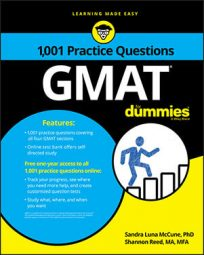Some of the Data Sufficiency questions in the GMAT Quantitative section will test your basic math skills, so you should brush up on your fractions, decimals, ratios and proportions, percent, and exponents.

Each Data Sufficiency problem poses a question, followed by two statements. Your task is to evaluate the statements to determine at what point there is or is not sufficient information to answer the question.

Unlike the Problem Solving questions, you do not actually have to answer the question posed. Instead, you select one of five fixed answer choices that offer different options about the sufficiency of the information provided in the two statements.

## Practice questions

1. A garden contains 32 tomato plants. How many pepper plants does the garden contain?

(1) The ratio of the number of tomato plants to the number of pepper plants is 8 to 3.

(2) If the number of tomato plants is increased by 4, and the number of pepper plants stays the same, the ratio of the number of tomato plants to the number of pepper plants is 3 to 1.

A. Statement (1) ALONE is sufficient, but statement (2) alone is not sufficient to answer the question asked.

B. Statement (2) ALONE is sufficient, but statement (1) alone is not sufficient to answer the question asked.

C. Both statements TOGETHER are sufficient, but NEITHER statement ALONE is sufficient to answer the question asked.

E. Statements (1) and (2) TOGETHER are NOT sufficient to answer the question asked.

2. If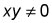what is the ratio of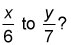(1) 7x = 6y

(2) x = 6

A. Statement (1) ALONE is sufficient, but statement (2) alone is not sufficient to answer the question asked.

B. Statement (2) ALONE is sufficient, but statement (1) alone is not sufficient to answer the question asked.

C. Both statements TOGETHER are sufficient, but NEITHER statement ALONE is sufficient to answer the question asked.

E. Statements (1) and (2) TOGETHER are NOT sufficient to answer the question asked.

1. The correct answer is D.

Let P = the number of pepper plants in the garden. Using the question information and (1) gives the proportion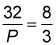which you can solve for P. Thus, (1) is sufficient.

Using the question information and (2) gives the proportion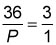which you can solve for P. Therefore, each statement alone is sufficient.

2. The correct answer is A.

The ratio of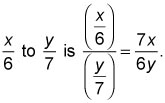From (1), 7x = 6y implies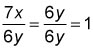Thus (1) is sufficient.

From (2), x = 6 implies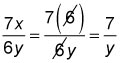The value of this expression varies depending on the value of y. Thus, (2) is not sufficient. Therefore, statement (1) alone is sufficient.